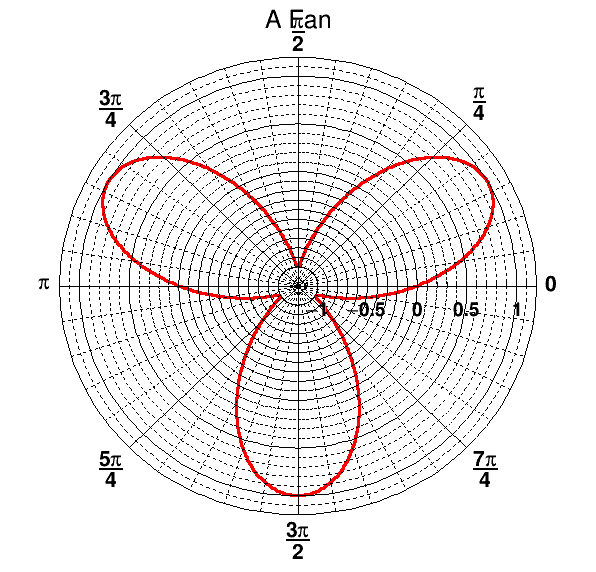# Building a polar graph in a square Canvas¶

Ported to nootbook by: Theis Hansen

In :
from ROOTaaS.iPyROOT import ROOT
ROOT.toCpp()

Welcome to ROOTaas Beta

Notebook is in Cpp mode


### The Graph¶

We create a new canvas using TCanvas. We then set rmin to 0 and rmax to the value of Pi times 6, the latter is done by calling on ROOT's mathematical toolkit using TMath. We state the number of points that we would like on the graph. We then write a loop too construct the graph. And we set the title, line width, color finally and we draw it!

In :
TCanvas c("myCanvas","myCanvas",600,600);
Double_t rmin=0.;
Double_t rmax=TMath::Pi()*6.;
const Int_t npoints=1000;
Double_t r[npoints];
Double_t theta[npoints];
for (Int_t ipt = 0; ipt < npoints; ipt++) {
r[ipt] = ipt*(rmax-rmin)/npoints+rmin;
theta[ipt] = TMath::Sin(r[ipt]);
}
TGraphPolar grP1 (npoints,r,theta);
grP1.SetTitle("A Fan");
grP1.SetLineWidth(3);
grP1.SetLineColor(2);

grP1.DrawClone("L");In [ ]: